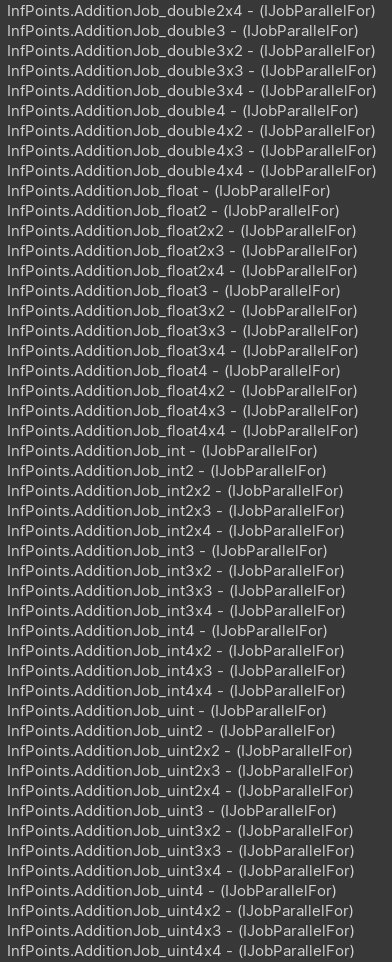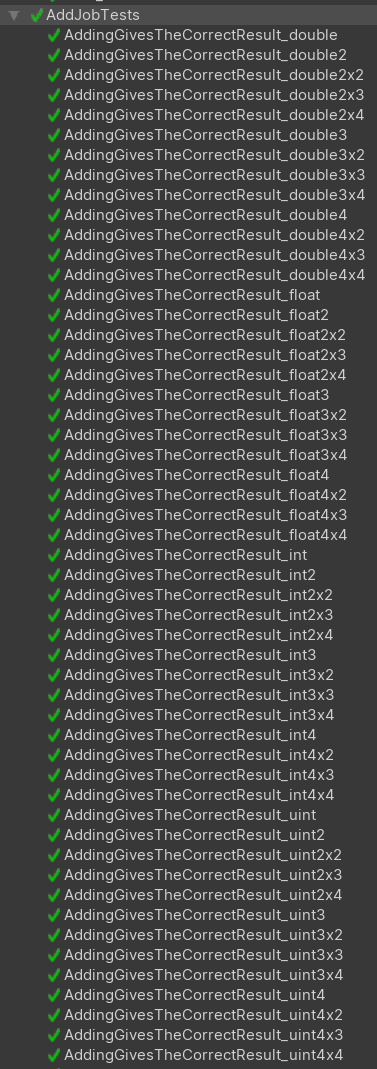You have a NativeArray of floats and and want to add a number to each element. You make an addition job. Easy!

``````[BurstCompile(FloatPrecision.Standard, FloatMode.Fast, CompileSynchronously = true)]
{
public NativeArray<float> Values;

public void Execute(int index)
{
}
}
``````

Then you do a quick test and realise that using `float4`s in the job instead of `floats` will allow Burst to use SIMD operations. This cuts the time to add to four million elements to almost a third (from 1.996ms to 0.765ms). So you copy and paste the job for a `float4` version. Later in the project you realise that `int` and `int4` version would be useful as well. So calling on the Rule of Three, you decide to generalise somehow.

You’d like to make jobs for each Unity.Mathematics numeric types; `double`, `float`, `int` and `uint` with the dimensions up to 4. This ends up being a lot of jobs! Unfortunately you can’t use Generics because there is no addition constraint. So the only real alternative is to use T4 text generation.

T4 templates let you write C# code to generate text. You may have noticed that the Unity.Mathematics code is all generated by T4 templates. And Unity.Collections is using T4 text generation to generate types for FixedList and NativeString.

So to generate jobs for each numeric type and each dimension I can use this template code:

``````<#
var TYPES = new []{"double","float","int","uint"};
foreach (var TYPE in TYPES)
{
for (int i = 1; i <= 4; i++)
{
for (int j = 1; j <= 4; j++)
{
string NUM1 = i==1 ? "" : i.ToString();
if (i == 1 && j > 1) break;
string SEP = j==1 ? "" : "x";
string NUM2 = j==1 ? "" : j.ToString();
var TYPE_FULL = \$"{TYPE}{NUM1}{SEP}{NUM2}";
#>
``````

and then the job looks like:

``````[BurstCompile(FloatPrecision.Standard, FloatMode.Fast, CompileSynchronously = true)]
{
public NativeArray<<#=TYPE_FULL#>> Values;

public void Execute(int index)
{
}
}
``````

Running it gives you all the combinations you need.You can even use the text templates to generate the tests. This may seem like a waste of test time, but it actually helped me find precision differences between the `float` and `float4` versions of these jobs.Very handy. I’m sure I’m going to be using more T4 templates in the future.

Resources